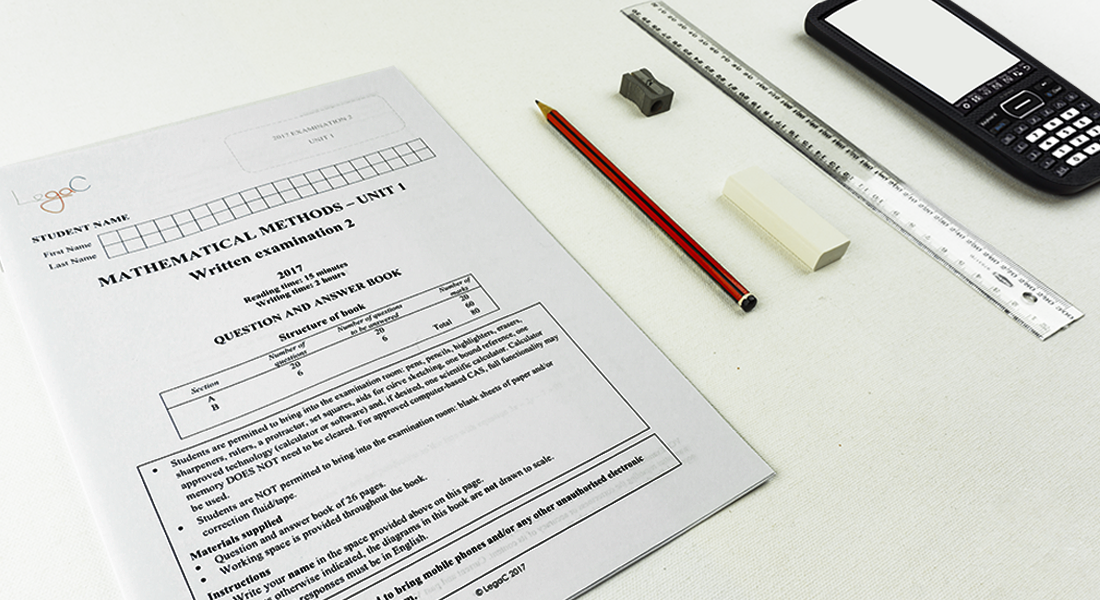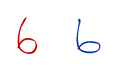## The exam is here... let's get started... with some tips

Authored By Carmen Popescu-Rose 10 Comment(s)
Once you have the exam paper in front of you, the first thing to do is ... take a few deep breaths and calm down ... It is not the end of the world. Just do your best.

1. READING TIME ... WHO NEEDS IT?

Well ... you do ... and here are some tips of using the reading time effectively.

• Read all the instructions given and make sure you have a good understanding of whether you have to answer all the sections in the paper or only some of them.
This applies to the Further Mathematics examinations where you have to answer two modules out of four.• If the paper has more than one section, check the total number of marks allocated to each section and divide the time accordingly.
The Specialist Mathematics written examination 2, for example, has two sections: Section A (20 marks) and Section B (60 marks), to be completed in 2 hours. This means that you should spend approximately 30 minutes on Section A and approximately 1.5 hours on Section B.Look through all the questions and make a mental note of the questions on three levels: 'easy', 'medium' and 'hard'. Keep in mind that the examinations are designed to test what you know. So, you need to start with the questions you know best.

2. WRITING TIME ... IT'S REALLY HERE

Wow ... what now?

• Check the number of marks allocated to the question.
1 mark questions require the correct answer only (a numerical answer or a brief and clear explanation).

2 mark questions require the correct method - method mark - and the correct answer - answer mark.

3 mark questions require the correct method - method mark, the correct answer - answer mark, and a third mark could be either a method mark or an answer mark for an intermediate step.

• Do not waste precious time ... every second counts (literally)!
Many students tend to write every single step and a lot of detail for questions that are worth 1 mark when it is not necessary.• Answer the question in the format required
Scientific notation or number of decimal places or exact form.

When you think you have answered the question, go back and read the question again to ensure that you have actually answered it. Often questions require a couple of steps to obtain the final answer. When students do not read the question properly, they stop half-way through the question giving an intermediate answer rather than the final answer.

• Sequential questions are related.
Many students do not realise that questions with parts iii, ... are quite often related to each other. Always check whether the answer to part i can be used in part ii. You will be surprised to see how often that happens.

Quite often, questions that have a 'Show that ... ' as part a or part i, use the answer in the next parts of the question.

• Use legible writing
Ensure that your handwriting is clear and does not require the examiner to guess your answer. The examiner is not going to guess or interpret your answer. They are going to read it as it shows.
Which one of the symbols below represents the number 6 and which one represents the small letter b?• Use of calculator when allowed
Use the calculator only if required. You should know, for example, that 2 ✕ 3 = 6 and other basic operations; do not waste time using a calculator for such operations.

• Rounding off should only be done on the final answer.
Set the calculator on degrees or radians as required by the question.

• Ensure that the answer makes sense
If your answer is something like: "The speed of a snail is 35 km/h", use common sense ... something does not add up here. Exam questions are written so that the answers are realistic values.

This is basically it ... study well and GOOD LUCK!wKCrlqyuxwdpGVimKWnuzMCAINpQvdqetFhUgZOkPoXTvqKltOnwWRkIvePwuGkmXfpENlIizoLfYDAHrKLzdUImOARGEWQXgPZDVBrpCfVdWvE